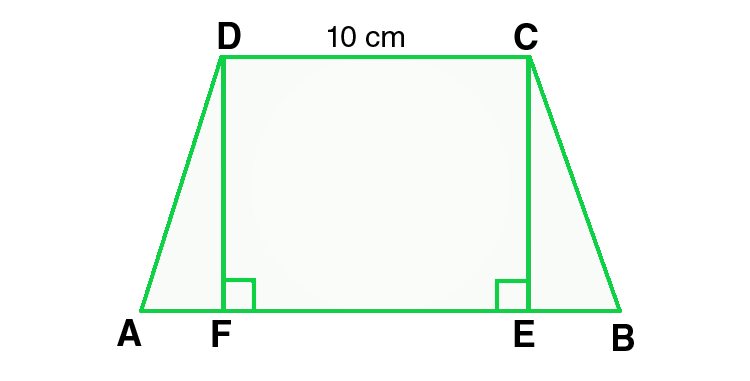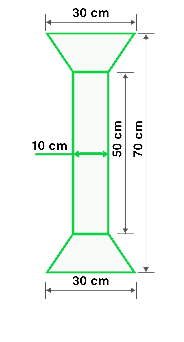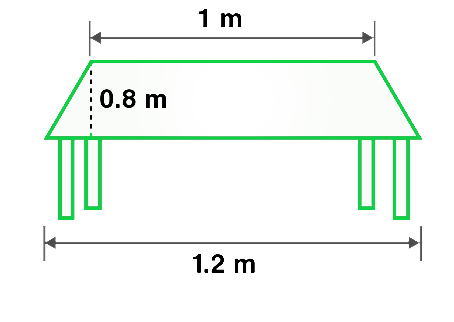GeeksforGeeks App
Open AppBrowser
Continue

## Related Articles

• RD Sharma Class 8 Solutions for Maths

# Class 8 RD Sharma Solutions – Chapter 20 Area Of Trapezium And Polygon- Exercise 20.2 | Set 1

### Question 1. Find the area, in square meters, of the trapezium whose bases and altitudes are as under :

(i) bases = 12 dm and 20 dm, altitude = 10 dm

(ii) bases = 28 cm and 3 dm, altitude = 25 cm

(iii) bases = 8 m and 60 dm, altitude = 40 dm

(iv) bases = 150 cm and 30 dm, altitude = 9 dm

Solution:

Area of trapezium = 1/2 (Sum of lengths of parallel sides) × altitude

(i) Given:

Length of bases of trapezium = 12 dm and 20 dm,

Length of altitude = 10 dm

As we know that 10 dm = 1 m

Therefore, Length of bases in m is 1.2 m and 2 m.

Similarly, length of altitude in m is 1 m.

Now, put the values in the formula(i.e. Area of trapezium),

Area of trapezium = 1/2 (1.2 + 2.0) × 1 =  1/2 × 3.2 = 1.6

Hence, Area of trapezium = 1.6m2

(ii) Given:

Length of bases of trapezium = 28 cm and 3 dm,

Length of altitude = 25 cm,

As we know that 10 dm = 1 m

Therefore, Length of bases in m = 0.28 m and 0.3 m

and length of altitude in m = 0.25 m

Now, put the values in the given formula(i.e. Area of trapezium),

Area of trapezium = 1/2 (0.28 + 0.3) × 0.25 = 1/2 × 0.58× 0.25 = 0.0725

Hence, Area of trapezium = 0.0725m2

(iii) Given:

Length of bases of trapezium = 8 m and 60 dm,

Length of altitude = 40 dm.

As we know that 10 dm = 1 m

Therefore, Length of bases in m = 8 m and 6 m

and length of altitude in m = 4 m

Now, put the values in the given formula(i.e. Area of trapezium),

Area of trapezium = 1/2 (8 + 6) × 4 = 1/2 × 56 = 28

Hence, Area of trapezium = 28m2

(iv) Given:

Length of bases of trapezium = 150 cm and 30 dm,

Length of altitude = 9 dm.

As we know that 10 dm = 1 m

Therefore, Length of bases in m = 1.5 m and 3 m

and length of altitude in m = 0.9 m.

Now, put the values in the given formula(i.e. Area of trapezium),

Area of trapezium = 1/2 (1.5 + 3) × 0.9 = 1/2 × 4.5 × 0.9 = 2.025

Hence, Area of trapezium = 2.025m2

### Question 2. Find the area of a trapezium with base 15 cm and height 8 cm, if the side parallel to the given base is 9 cm long.

Solution:

Given:

Length of bases of trapezium = 15 cm and 9 cm,

Length of altitude = 8 cm.

As we know that,

Area of trapezium = 1/2 (Sum of lengths of parallel sides) × altitude

Now, put all the given values in this formula, and we get,

Area of trapezium = 1/2 (15 + 9) × 8 = 1/2 × 192 = 96

Hence, the area of trapezium = 96m2

### Question 3. Find the area of a trapezium whose parallel sides are of length 16 dm and 22 dm and whose height is 12 dm.

Solution:

Given:

Length of bases of trapezium = 16 dm and 22 dm,

Length of altitude = 12 dm.

As we know that 10 dm = 1 m

Therefore, the length of bases in m = 1.6 m and 2.2 m

and length of altitude in m = 1.2 m.

As we know that,

Area of trapezium = 1/2 (Sum of lengths of parallel sides) × altitude

Now, put all the given values in this formula, and we get,

Area of trapezium = 1/2 (1.6 + 2.2) × 1.2 = 1/2 × 3.8 × 1.2 = 2.28

Hence, the Area of trapezium = 2.28m2

### Question 4. Find the height of a trapezium, the sum of the lengths of whose bases (parallel sides) is 60 cm and whose area is 600 cm2.

Solution:

Given:

Length of bases of trapezium = 60 cm,

Area = 600 cm2

As we know that,

Area of trapezium = 1/2 (Sum of lengths of parallel sides) × altitude

Now, put all the given values in this formula, and we get,

600 = 1/2 (60) × altitude

600 = 30 × altitude

altitude = 600/30 = 20

Hence, the length of altitude is 20 cm.

### Question 5. Find the altitude of a trapezium whose area is 65 cm2 and whose base is 13 cm and 26 cm.

Solution:

Given:

Length of bases of trapezium = 13 cm and 26 cm,

Area = 65 cm2

As we know that,

Area of trapezium = 1/2 (Sum of lengths of parallel sides) × altitude

Now, put all the given values in this formula, and we get,

65 = 1/2 (13 + 26) × altitude

65 = 39/2 × altitude

altitude = (65×2) /39 = 130/39 = 10/3

Hence, the length of altitude = 10/3 cm

### Question 6. Find the sum of the lengths of the bases of a trapezium whose area is 4.2 m2 and whose height is 280 cm.

Solution:

Given:

Height of trapezium = 280 cm = 2.8m,

Area = 4.2 m2,

As we know that,

Area of trapezium = 1/2 (Sum of lengths of parallel sides) × altitude

We can also rewrite the formula as,

Sum of lengths of parallel sides = (2 × Area) / altitude

= (2 × 4.2) / 2.8 = 8.4/2.8 = 3

Hence, the sum of lengths of parallel sides = 3 m

### Question 7. Find the area of a trapezium whose parallel sides of lengths 10 cm and 15 cm are at a distance of 6 cm from each other. Calculate this area as,

(i) The sum of the areas of two triangles and one rectangle.

(ii) The difference of the area of a rectangle and the sum of the areas of two triangles.

Solution:(i) As we know that,

Area of a trapezium ABCD = area of ∆DFA + area of rectangle(DFEC) + area of ∆CEB

= (1/2 × AF × DF) + (FE × DF) + (1/2 × EB × CE)

= (1/2 × AF × h) + (FE × h) + (1/2 × EB × h)

= 1/2 × h × (AF + 2FE + EB) = 1/2 × h × (AF + FE + EB + FE)

= 1/2 × h × (AB + FE)

= 1/2 × h × (AB + CD)           -(Opposite sides of rectangle are equal)

= 1/2 × 6 × (15 + 10) = 1/2 × 6 × 25 = 75

Hence, the area of trapezium = 75 cm2

(ii) Area of rectangle ABEF = 15 x 6 = 90 cm2

Area of ∆ADF + ∆BEC = 1/2 (FD + CE) x DM

= 1/2 x (15 – 10) x 6 = 1/2 x 5 x 6 = 15 cm2

Area of trapezium = 90 – 15 = 75 cm²           -(Area of rectangle – Area of two triangles)

Hence the difference of the area of a rectangle and

the sum of the areas of two triangles is 75 cm2

### Question 8. The area of a trapezium is 960 cm2. If the parallel sides are 34 cm and 46 cm, find the distance between them.

Solution:

As we know that,

Area of trapezium = 1/2 (Sum of lengths of parallel sides) × distance between parallel sides(height)

Also, we can rewrite the formula as,

Distance between parallel sides = (2 × Area) / Sum of sides

Now, put all the given values in this formula, and we get,

= (2 × 960) / (34 + 46) = (2 × 960) / 80 = 1920/80 = 24

Hence, the distance between parallel sides = 24 cm

### Question 9. Find the area of Fig. as the sum of the areas of two trapeziums and a rectangle.Solution:

Given:

Length of rectangle = 50 cm

Breadth of rectangle = 10 cm

Length of the parallel sides of the trapezium = 30 cm and 10 cm

Distance between parallel sides of trapezium = (70 – 50)/2 = 20/2 = 10

So, the height of the trapezium is 10cm

From the given figure we conclude that,

Area of figure = Area of two trapeziums + Area of rectangle

= 2 × 1/2 (Sum of lengths of parallel sides) × altitude + Length × Breadth

Now, put all the given values in this formula, and we get,

= 2 × 1/2 (30+10) × 10 + 50 × 10 = 40 × 10 + 50 × 10

= 400 + 500 = 900

Hence, the area of figure = 900 cm2

### Question 10. Top surface of a table is trapezium in shape. Find its area if its parallel sides are 1 m and 1.2 m and the perpendicular distance between them is 0.8 m.Solution:

Given:

Length of the parallel sides of the trapezium = 1.2m and 1m,

Distance between parallel sides of trapezium(height) = 0.8m

As we know that,

Area of trapezium = 1/2 (Sum of lengths of parallel sides) × distance between parallel sides

Now, put all the given values in this formula, and we get,

Area of trapezium = 1/2 (1.2 + 1) × 0.8 = 1/2 × 2.2 × 0.8 = 0.88

Hence, the area of trapezium = 0.88m2

### Chapter 20 Area Of Trapezium And Polygon- Exercise 20.2 | Set 2

My Personal Notes arrow_drop_up
Related Tutorials